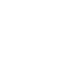## Common Ratios

2/1 = .5, 3/2 = 1.5, 5/3 = 1.666, 8/5 = 1.6, 13/8 = 1.62, 21/13 = 1.615, 34/21 = 1.619, 55/34 = 1.6176, 89/55 = 1.61818, 144/89 = 1.61797

Math is an exact science, carried out to infinite sequencing, such as pi. The same is true for the Fibonacci sequence of numbers. The ratios above are expressions of lengths to widths that have eventually been called the Golden Mean, or Divine Ratio. (The curious thing is that as the ratios continue, the “Golden Mean” approaches the mathematical formula of the Fibonacci Sequence. They are related!)

Supposedly these ratios create a rectangle that is the most pleasing to the natural eye, so that compositions in art or architecture are said to have these ratios imbedded in them. To a certain extant this may be true.

Classic photography has taught the rule of thirds for decades upon decades. Then, the more advanced courses modified it to 8/5s. Basically meaning that the best division of a rectangle is to divide it into 1/8s and then draw a line at 3/8s or 5/8s and that gives the “best” dividing line. The ratio is 1.6, and the most accurate Fibonacci sequence is 1.61797. We used to that this is “Good enough for government work”.

The “Golden Mean” and the “Fibonacci Sequence” are said to be a part of a standard composition practice. It may be true in a sense. We compose things to patterns that are most familiar. Because these ratios are so similar in so many images and objects, we are certain to find them as ongoing patterns in our own work. But in general, the exact nature of the mathematical basis is rarely found in art or architecture, approximations yes, exact no.

The one area that appears to have the most accuracy is in nature. The pattern of leaves and seed pods seem to reflect the formula with the most accuracy, far more than random chance. Unfortunately, the most famous example of the appearance in nature of the Fibonacci Sequence is the Nautilus Sea Shell, and that probably is not true. It is a spiral but not as exact as other more naturally occurring Fibonacci spirals. The controlling factor appears to be rain and sunlight. The Fibonacci Sequence give the optimum distancing to give each leaf/seed the most exposure.

So why do we see this ratio in art/architecture? It most certainly has been intentionally used in some cases. Mostly, the ratio we see is the “learned patterned”. We see repeated ratios of television/monitors, magazines, architectural facades. Sometimes they are so close to 1.6 we just assume that it was intentional. In point of fact, there isn’t a “perfect rectangle”, only the most observed rectangle.

Even still, I intentionally use the 8/5s ratio in my photographs and my artwork (when I remember that I can, hehe)